# Breadth First Search in Python (with Code) | BFS Algorithm

Breadth-first search and Depth-first search in python are algorithms used to traverse a graph or a tree. They are two of the most important topics that any new python programmer should definitely learn about. Here we will study what breadth-first search in python is, understand how it works with its algorithm, implementation with python code, and the corresponding output to it. Also, we will find out the application and uses of breadth-first search in the real world.

As discussed earlier, Breadth-First Search (BFS) is an algorithm used for traversing graphs or trees. Traversing means visiting each node of the graph. Breadth-First Search is a recursive algorithm to search all the vertices of a graph or a tree. BFS in python can be implemented by using data structures like a dictionary and lists. Breadth-First Search in tree and graph is almost the same. The only difference is that the graph may contain cycles, so we may traverse to the same node again.

## BFS Algorithm

Before learning the python code for Breadth-First and its output, let us go through the algorithm it follows for the same. We can take the example of Rubik’s Cube for the instance. Rubik’s Cube is seen as searching for a path to convert it from a full mess of colors to a single color. So comparing the Rubik’s Cube to the graph, we can say that the possible state of the cube is corresponding to the nodes of the graph and the possible actions of the cube is corresponding to the edges of the graph.

As breadth-first search is the process of traversing each node of the graph, a standard BFS algorithm traverses each vertex of the graph into two parts: 1) Visited 2) Not Visited. So, the purpose of the algorithm is to visit all the vertex while avoiding cycles.

BFS starts from a node, then it checks all the nodes at distance one from the beginning node, then it checks all the nodes at distance two, and so on. So as to recollect the nodes to be visited, BFS uses a queue.

The steps of the algorithm work as follow:

1.  Start by putting any one of the graph’s vertices at the back of the queue.
2.  Now take the front item of the queue and add it to the visited list.
3.  Create a list of that vertex's adjacent nodes. Add those which are not within the visited list to the rear of the queue.
4.  Keep continuing steps two and three till the queue is empty.

Many times, a graph may contain two different disconnected parts and therefore to make sure that we have visited every vertex, we can also run the BFS algorithm at every node.

### BFS pseudocode

The pseudocode for BFS in python goes as below:

create a queue Q

mark v as visited and put v into Q

while Q is non-empty

remove the head u of Q

mark and enqueue all (unvisited) neighbors of u

## BFS implementation in Python (Source Code)

Now, we will see how the source code of the program for implementing breadth first search in python.

Consider the following graph which is implemented in the code below: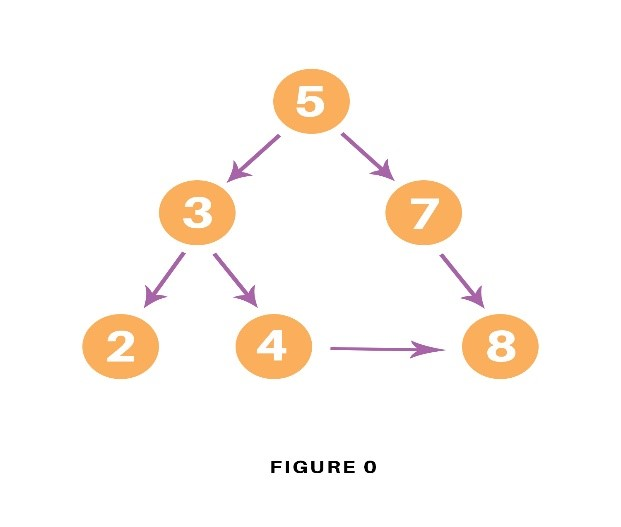```graph = {
'5' : ['3','7'],
'3' : ['2', '4'],
'7' : ['8'],
'2' : [],
'4' : ['8'],
'8' : []
}

visited = [] # List for visited nodes.
queue = []     #Initialize a queue

def bfs(visited, graph, node): #function for BFS
visited.append(node)
queue.append(node)

while queue:          # Creating loop to visit each node
m = queue.pop(0)
print (m, end = " ")

for neighbour in graph[m]:
if neighbour not in visited:
visited.append(neighbour)
queue.append(neighbour)

# Driver Code
bfs(visited, graph, '5')    # function calling
```

In the above code, first, we will create the graph for which we will use the breadth-first search. After creation, we will create two lists, one to store the visited node of the graph and another one for storing the nodes in the queue.

After the above process, we will declare a function with the parameters as visited nodes, the graph itself and the node respectively. And inside a function, we will keep appending the visited and queue lists.

Then we will run the while loop for the queue for visiting the nodes and then will remove the same node and print it as it is visited.

At last, we will run the for loop to check the not visited nodes and then append the same from the visited and queue list.

As the driver code, we will call the user to define the bfs function with the first node we wish to visit.

### Output

The output of the above code will be as follow:

5 3 7 2 4 8

### Example

Let us see how this algorithm works with an example. Here, we will use an undirected graph with 5 vertices.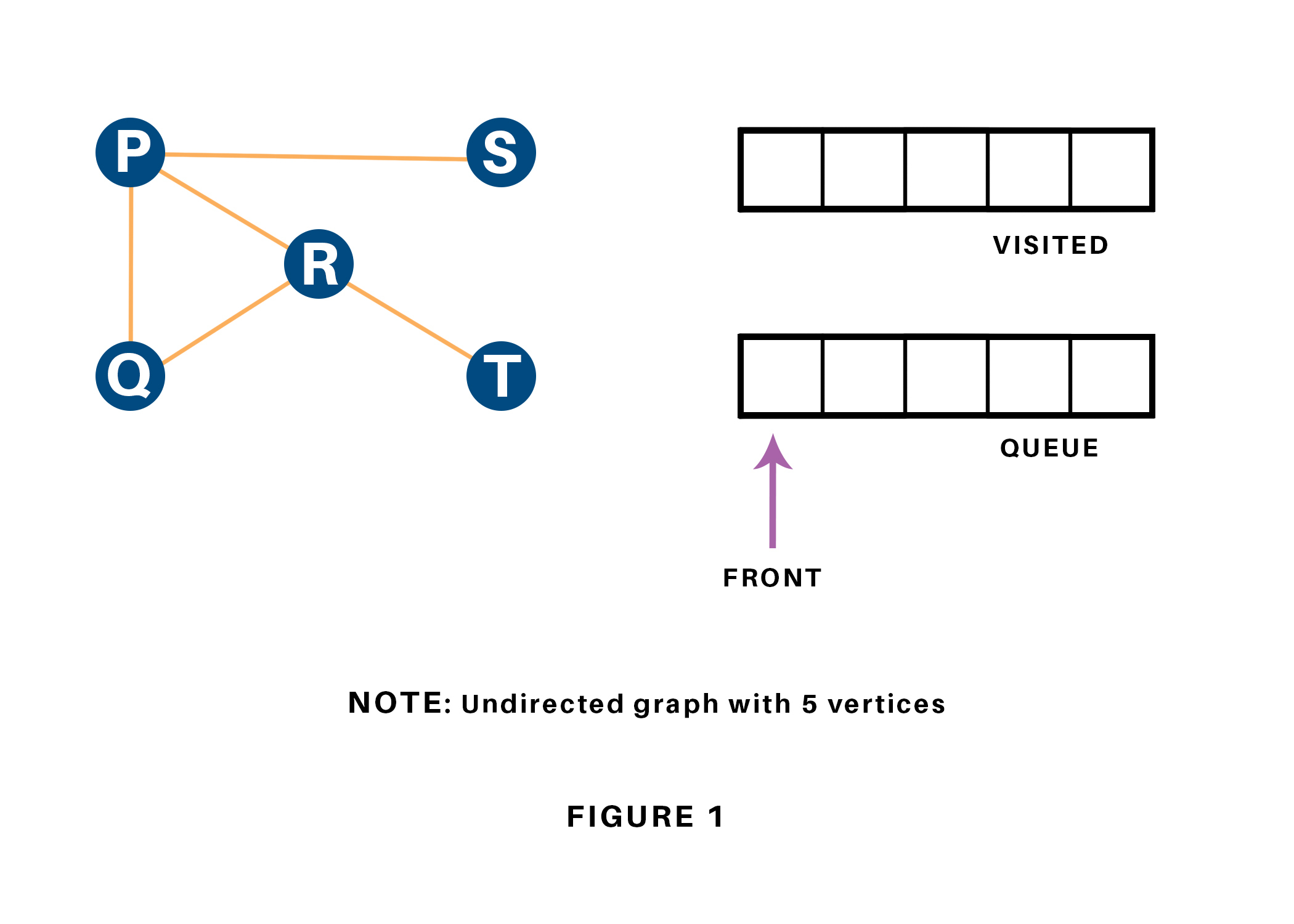We begin from the vertex P, the BFS algorithmic program starts by putting it within the Visited list and puts all its adjacent vertices within the stack.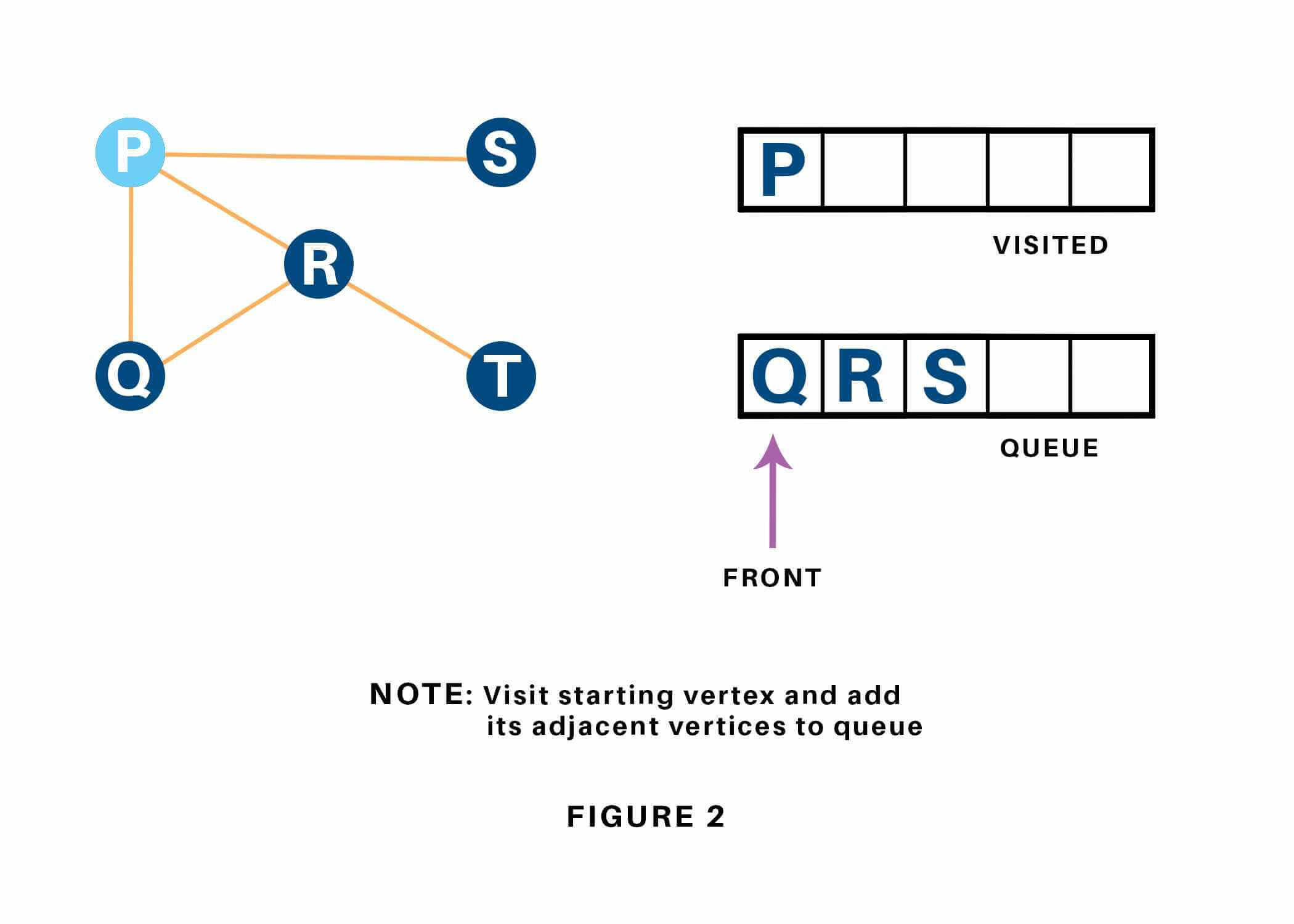Next, we have a tendency to visit the part at the front of the queue i.e. Q and visit its adjacent nodes. Since P has already been visited, we have a tendency to visit R instead.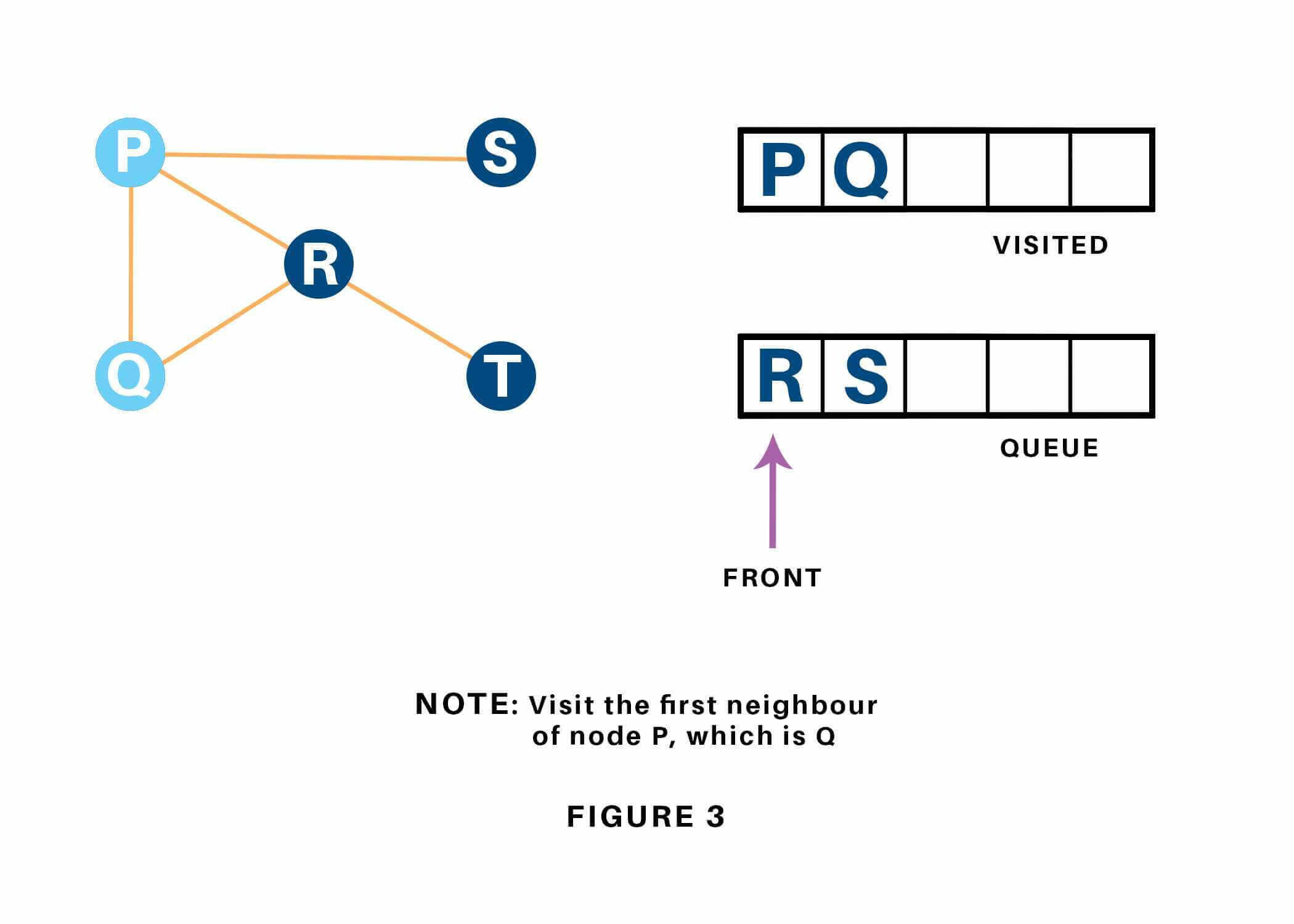Vertex R has an unvisited adjacent vertex in T, thus we have a tendency to add that to the rear of the queue and visit S, which is at the front of the queue.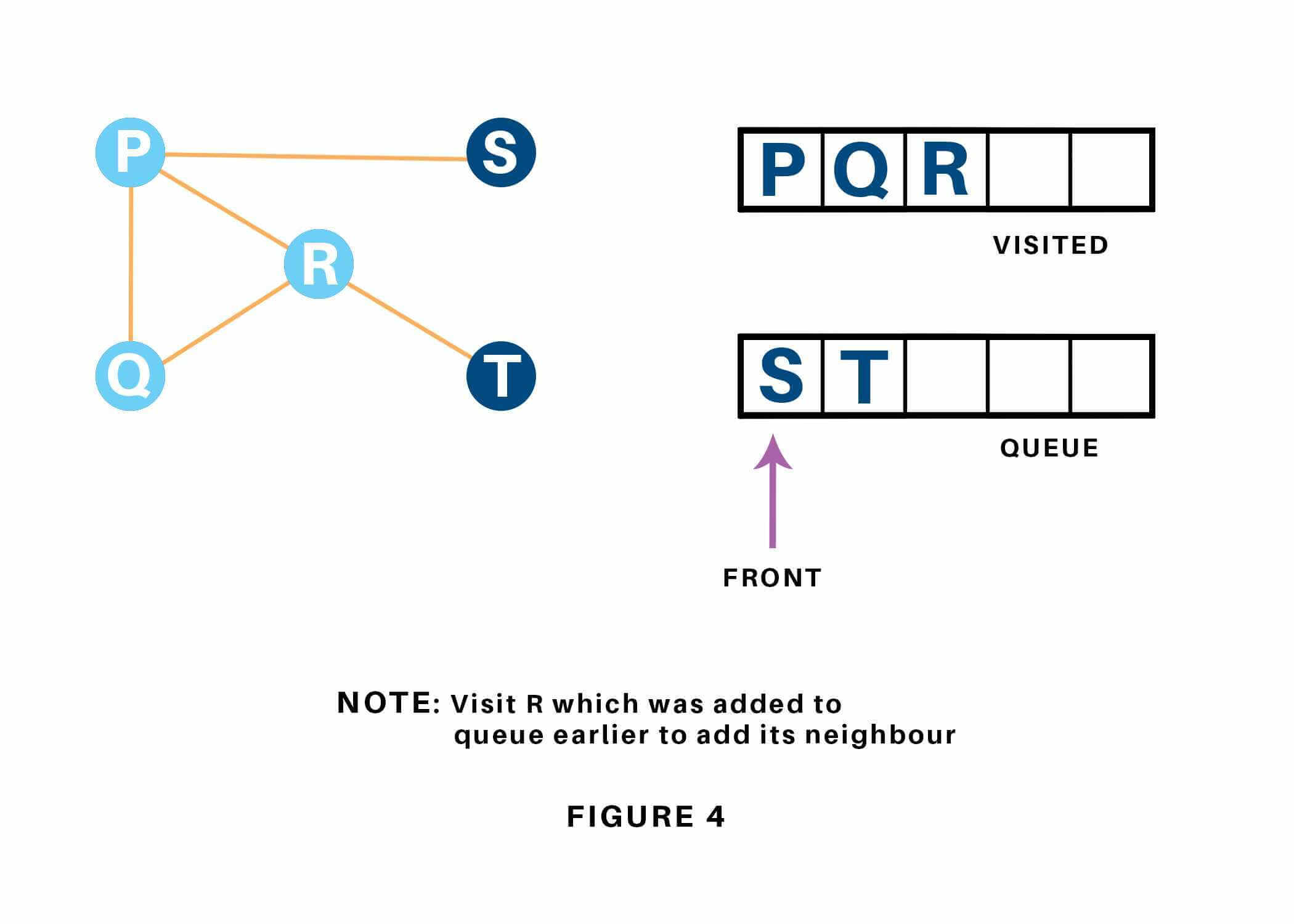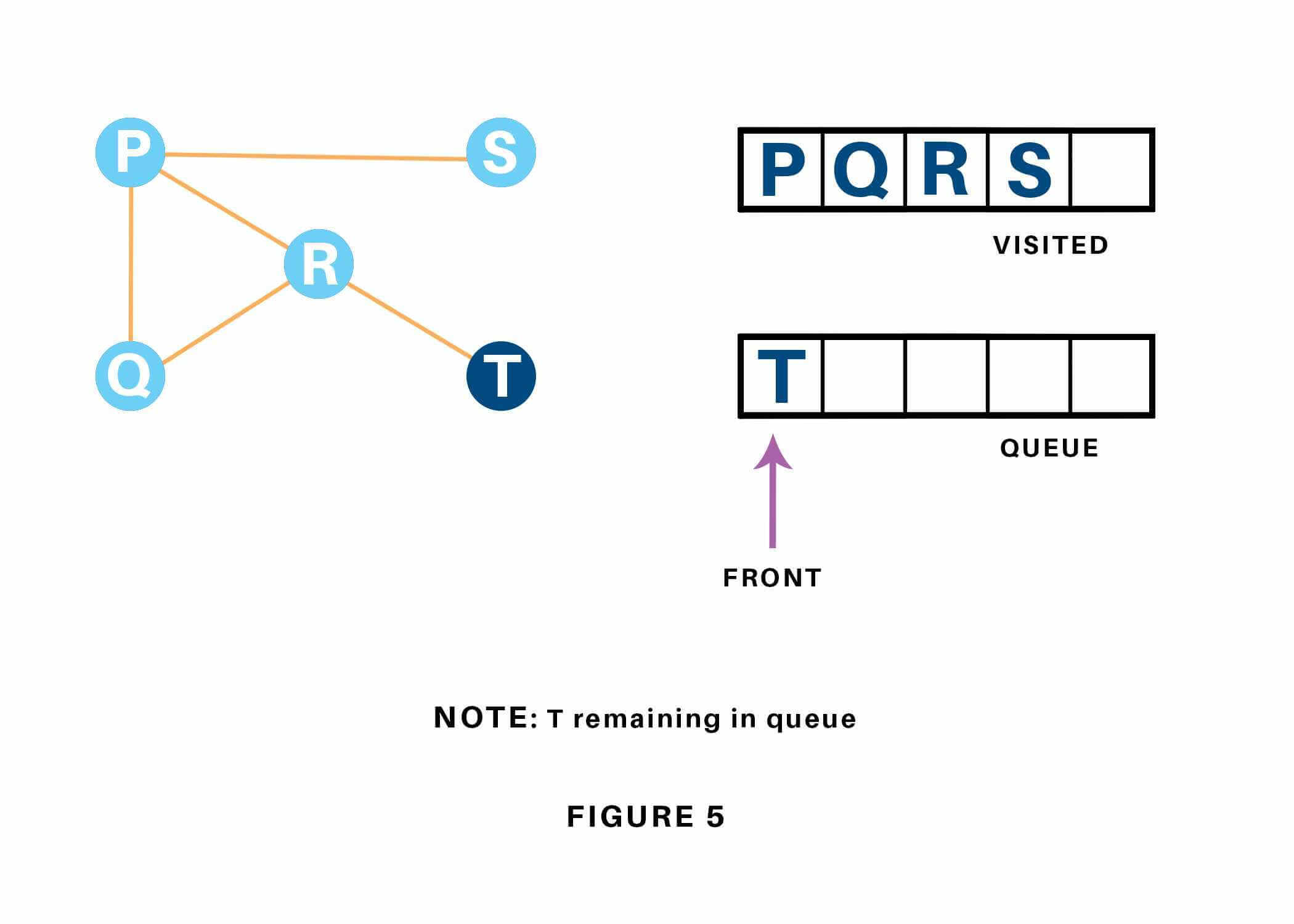Now, only T remains within the queue since the only adjacent node of S i.e. P is already visited. We have a tendency to visit it.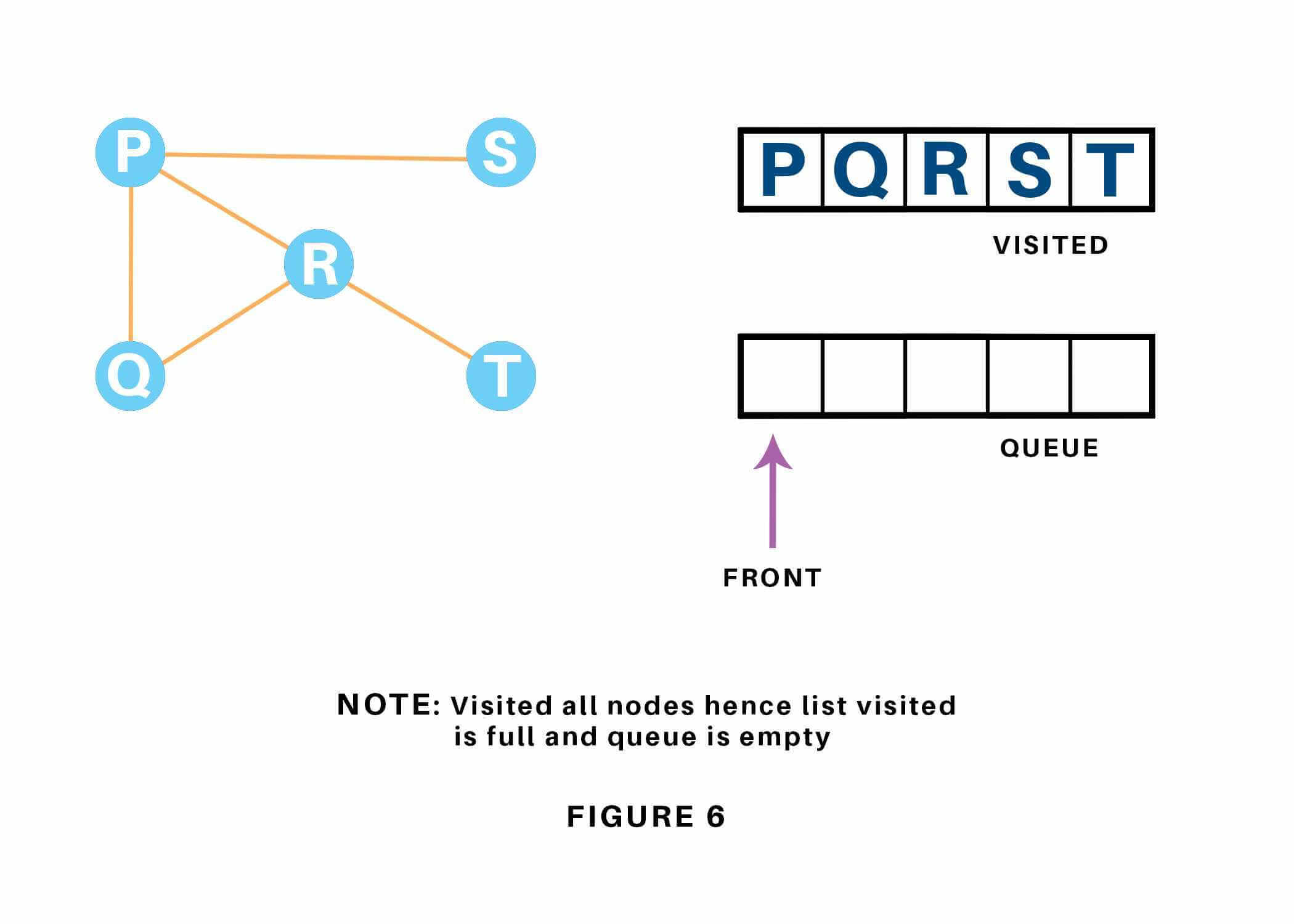Since the queue is empty, we've completed the Traversal of the graph.

### Time Complexity

The time complexity of the Breadth first Search algorithm is in the form of O(V+E), where V is the representation of the number of nodes and E is the number of edges.

Also, the space complexity of the BFS algorithm is O(V).

### Applications

Breadth-first Search Algorithm has a wide range of applications in the real-world. Some of them are as discussed below:

1.  In GPS navigation, it helps in finding the shortest path available from one point to another.
2.  In pathfinding algorithms
3.  Cycle detection in an undirected graph
4.  In minimum spanning tree
5.  To build index by search index
6.  In Ford-Fulkerson algorithm to find maximum flow in a network.

## Conclusion

If you have a good understanding of core python concepts and with the help of what you read today, you can now easily implement breadth first search in python. I hope this article clearly explained how this algorithm works.  If you need help with Python homework, contact our experts now!

### FavTutor - 24x7 Live Coding Help from Expert Tutors!